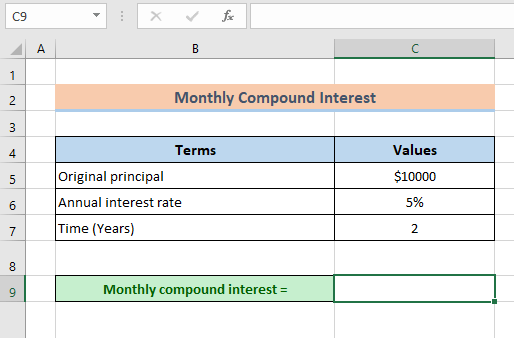# How To Calculate Compound Interest In Excel

Calculating compound interest is a crucial task that most financial investors need to know. Unlike simple interest, compound interest can earn more money at the end of the borrowing or investment period.

Compound interest constitutes the original interest and all interests charged on the accrued principal value. To get compound interest for an investment, an investor needs to add the original principal to the accrued interest before calculating interest earned in the next payment period.

It is also called monthly compound interest when the interest is calculated after each month for 12 months in a year. You can calculate the compound interest using the basic mathematical general formula: I = PV(1+r)n – PV, where FV is the future value, PV is the present value, r is the interest charged per period, and n is the number of the period the interest is compounded. However, Excel allows you to calculate monthly compound interest for investment by using other formulas, as discussed below.

## Using The Basic Formula to Calculate Monthly Compound Interest

1. Assuming Cell C5 contains the original principal or present value (PV), C6 contains the annual rate, and C7 contains time in years. Here, you will multiply the original principal by the interest rate charged. In this case, you can type the formula below in any cell, such as cell C9.=C5*

2. Since the interest is compounded monthly, you must divide the annual interest rate by 12. The next formula will look like this:

=C5*(1+(C6/12))3. Now that you have the rate compounded 12 times in a year, type the cell reference in which time is given in years. Then, multiply it by 12, which gives the next formula:

=C5*(1+(C6/12))^(12*C7)4. Subtract the principal amount from the formula whose cell reference is C5 to get your compound interest. The final formula will be:

=C5*(1+(C6/12))^(12*C7)-C55. Press the Enter button to complete the calculation.## Using Excel FV Function

The FV function gives you the future value of an investment value. Its generic formula is:

=FV(rate,nper,pmt,[pv],[type]), where;

rate is interest charged per period.

nper is the total payment period.

pmt specifies payment per period (optional).

[pv] specifies the present value of the investment (optional). If the present value is not given, you can leave it blank, and Excel will default it to zero. However, if you omit PV, you must provide the pmt argument.

[type] specifies if the payment is made at the start or the end of the period (optional). If the payment is made at the start, you must type 1. On the contrary, if the payment is made at the end, you must type 0.

Steps:

1. Using the same example as the method above, you need to specify the rate in the FV function. Also, since you need to calculate the monthly compound, you must divide the annual rate by 12. Thus, type the following formula in any cell, such as C9:

=FV(C6/122. Since the payment period is given in years yet you need the compound interest in months, you have to multiply the time by 12. In this case, you will multiply the cell reference in which time is recorded by 12 as follows;

=FV(C6/12,C7*12

3. Next, since no amount is paid or added to the original principal value within the investment period, you will include 0 value for pmt. The next formula will look like this:

=FV(C6/12,C7*12,0,4. After omitting the pmt value, the cell reference with the original principal value (PV) will have a negative sign (-). Therefore, your formula will turn to:

=FV(C6/12,C7*12,0,-C5)

5. Lastly, to get monthly compound interest, subtract the original principal from the future value as follows:

=FV(C6/12,C7*12,0,-C5)-C56. Hit the Enter button.## Using the FVSCHEDULE Function

The FVSCHEDULE function also gives the future values of an investment, though with a variable interest rate. It is represented by the syntax:

=FVSCHEDULE(principal, schedule), where;

principal is the present value of an investment

schedule represents an array of values that provide a schedule of interest rates charged on the principal value.

1. First, since you need to calculate monthly compound interest, you must divide the annual rate by 12. Therefore, you can type the following formula in cell C9

=C6/122. In the next cell C10, insert the FVSCHEDULE function with the cell reference for the original principal as follows:

=FVSHEDULE(C5,3. Since the schedule argument in the function requires you to provide an array of interests charged on the original principal, you need to insert monthly interest 12 times in the formula. Your formula in cell C10 will look like this:

=FVSCHEDULE(C5,{O.OO5,O.005,0.005,0.005,0.005,0.005,0.005,0.005,0.005,0.005,0.005,0.005})

4. Now subtract the original principal from the future value to get the compound interest.

=FVSCHEDULE(C5,{O.005,0.005,0.005,0.005,0.0005,0.005,0.005,0.0005,0.005,0.005,0.005,0.005})-C5.

5. Hit the Enter button.